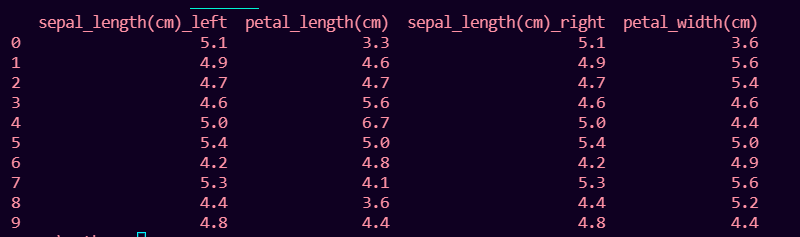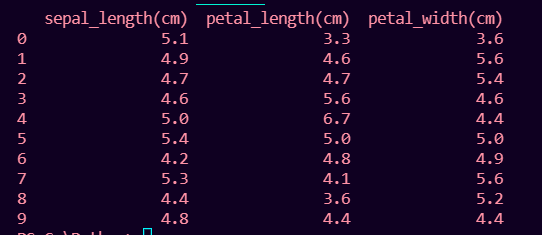GeeksforGeeks App
Open AppBrowser
Continue

# How to Fix: columns overlap but no suffix specified

In this article, we will fix the error: columns overlap but no suffix specified in Python.

This error occurs when we join two pandas data frames that have one or more same columns but there is no suffix specified to differentiate them.

Error:

ValueError: columns overlap but no suffix specified

## Python3

 `# importing pandas``import` `pandas as pd``# importing numpy``import` `numpy as np`` ` ` ` `sepal_length ``=` `[``5.1``, ``4.9``, ``4.7``, ``4.6``, ``5.0``,``                ``5.4``, ``4.2``, ``5.3``, ``4.4``, ``4.8``]``petal_length ``=` `[``3.3``, ``4.6``, ``4.7``, ``5.6``, ``6.7``, ``                ``5.0``, ``4.8``, ``4.1``, ``3.6``, ``4.4``]`` ` `# numpy array of length 7``petal_width ``=` `[``3.6``, ``5.6``, ``5.4``, ``4.6``, ``4.4``, ``               ``5.0``, ``4.9``, ``5.6``, ``5.2``, ``4.4``]`` ` `# DataFrame with 2 columns of length 10``df1 ``=` `pd.DataFrame({``'sepal_length(cm)'``: sepal_length,``                    ``'petal_length(cm)'``: petal_length})`` ` `df2 ``=` `pd.DataFrame({``'sepal_length(cm)'``: sepal_length,``                    ``'petal_width(cm)'``: petal_width})`` ` `print``(df1.join(df2))`

Output:

ValueError: columns overlap but no suffix specified: Index([‘sepal_length(cm)’], dtype=’object’)

### Reason for the error :

The data frames df1 and df2 have the common column sepal_length(cm) that is the intersection of two data frames is not empty. To check if there are any intersection columns :

`print(df1.columns.intersection(df2.columns))`

Output:

`Index(['sepal_length(cm)'], dtype='object')`

sepal_length(cm) is the intersection column here.

### Fixing the error:

This error can be fixed by using the join() or merge() method on the two data frames.

## Method1 : Using join() method

The suffixes should be given while using the join method to avoid this error. By default, it does inner join on two tables. This can be changed by mentioning the parameters in join() function.

Syntax:

`df1.join(df2, lsuffix='suffix_name', rsuffix='suffix_name')`

where

• df1 is the first dataframe
• df2 is the second dataframe

Example:

## Python3

 `# importing pandas``import` `pandas as pd``# importing numpy``import` `numpy as np`` ` ` ` `sepal_length ``=` `[``5.1``, ``4.9``, ``4.7``, ``4.6``, ``5.0``, ``5.4``,``                ``4.2``, ``5.3``, ``4.4``, ``4.8``]``petal_length ``=` `[``3.3``, ``4.6``, ``4.7``, ``5.6``, ``6.7``, ``5.0``,``                ``4.8``, ``4.1``, ``3.6``, ``4.4``]`` ` `# numpy array of length 7``petal_width ``=` `[``3.6``, ``5.6``, ``5.4``, ``4.6``, ``4.4``, ``5.0``,``               ``4.9``, ``5.6``, ``5.2``, ``4.4``]`` ` `# DataFrame with 2 columns of length 10``df1 ``=` `pd.DataFrame({``'sepal_length(cm)'``: sepal_length,``                    ``'petal_length(cm)'``: petal_length})`` ` `df2 ``=` `pd.DataFrame({``'sepal_length(cm)'``: sepal_length,``                    ``'petal_width(cm)'``: petal_width})`` ` `print``(df1.join(df2, lsuffix``=``'_left'``, rsuffix``=``'_right'``))`

Output:## Method 2:  Using merge() method

This merge() method considers the common column in the 2 data frames and drops the common column in one of the data frames.

Syntax:

```pd.merge(df1,df2, how='join_type', on=None, left_on= None,
right_on = None, left_index =boolean,
right_index=boolean, sort=boolean)```

where,

• df1 is the first dataframe
• df2 is the second dataframe

The type of joining and the names of left and right data frames common columns can also be mentioned as parameters in the merge() function.

Example:

## Python3

 `# importing pandas``import` `pandas as pd``# importing numpy``import` `numpy as np`` ` ` ` `sepal_length ``=` `[``5.1``, ``4.9``, ``4.7``, ``4.6``, ``5.0``, ``5.4``,``                ``4.2``, ``5.3``, ``4.4``, ``4.8``]``petal_length ``=` `[``3.3``, ``4.6``, ``4.7``, ``5.6``, ``6.7``, ``5.0``, ``                ``4.8``, ``4.1``, ``3.6``, ``4.4``]`` ` `# numpy array of length 7``petal_width ``=` `[``3.6``, ``5.6``, ``5.4``, ``4.6``, ``4.4``, ``5.0``, ``               ``4.9``, ``5.6``, ``5.2``, ``4.4``]`` ` `# DataFrame with 2 columns of length 10``df1 ``=` `pd.DataFrame({``'sepal_length(cm)'``: sepal_length,``                    ``'petal_length(cm)'``: petal_length})`` ` `df2 ``=` `pd.DataFrame({``'sepal_length(cm)'``: sepal_length,``                    ``'petal_width(cm)'``: petal_width})`` ` `print``(pd.merge(df1, df2))`

Output:My Personal Notes arrow_drop_up